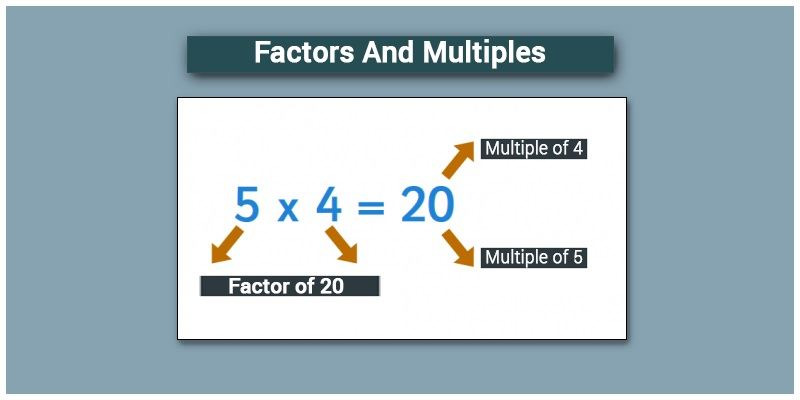Checkout JEE MAINS 2022 Question Paper Analysis : Checkout JEE MAINS 2022 Question Paper Analysis :

# Factors And Multiples

In the elementary level, the factors and multiples are the two key concepts which are studied together. Factors are the numbers which divide the given number exactly, whereas the multiples are the numbers which are multiplied by the other number to get specific numbers. It is time to recollect, to understand the concept of multiples and factors of a number. In this article, you will learn the detailed definition of factors and multiples with examples and the difference between them in detail.

## What are the Factors?

When a number is said to be a factor of any other second number, then the first number must divide the second number completely without leaving any remainder. In simple words, if a number (dividend) is exactly divisible by any number (divisor), then the divisor is a factor of that dividend. Every number has a common factor that is one and the number itself.For example, 4 is a factor of 24, i.e. 4 divides 24 exactly giving 6 as quotient and leaving zero as remainder. Alternatively, 6 is also a factor of 24 as it gives 4 as quotient on division. Therefore, 24 has 1, 24, 4, 6 as its factors in addition to 2, 3, 8 and 12 and all these numbers divide 24 exactly leaving no remainder.

If any natural number has only two factors, i.e. 1 and the number itself as its factors, such numbers are called prime numbers. 2 is an example of a prime number where it has only two factors, i.e. 1 and 2.

## What are Multiples?

A multiple of a number is a number that is the product of a given number and some other natural number. Multiples can be observed in a multiplication table. Multiples of some numbers are as follows:

Multiples of 2 are 2, 4, 6, 8, 10, 12, 14, 16, 18, 20, and so on Hence, multiples of 2 will be even numbers and will end with 0, 2, 4, 6 or 8.

Multiples of 3 are 3, 6, 9, 12, 15, 18, 21, and so on.

Multiples of 5 are 5, 10, 15, 20, 25, and so on.

Every multiple of 5 has its last digit as 0 or 5.

In the above-mentioned examples, say multiples of 2, the number 2 can be multiplied by infinite numbers to find the “n” number of multiples. Now, let us assume an example,

3 × 4 = 12

Here, 3 and 4 are the factors of 12,

12 is multiple of 3 and 4

Thus, we can conclude that if X and Y are two numbers and;

•    If X divides Y, X is a factor of Y

•    If Y is divisible by X, Y is a multiple of X

Since the number 1 divides every integer, it is a common factor of every integer. Also, every number is divisible by 1 and every number is a multiple of 1.

## Difference Between Factors and Multiples

The difference between factors and multiples are given here in tabular form. Go through the below table to understand the difference between them.

 S.No Factors Multiples 1 Factors are defined as the exact divisors of the given number The multiples are defined as the numbers obtained when multiplied by other numbers 2 The number of factors is finite The number of multiplies is infinite 3 The operation used to find the factors is a division The operation used to find the multiples is a multiplication 4 The outcome of the factors should be less than or equal to the given number The outcome of the multiples should be greater than or equal to the given number

### Factors and Multiples Examples

Example 1:

Find the factors of 20.

Solution:

The factors of 20 are 1, 2, 4, 5, 10 and 20.

Because the number 20 is exactly divisible by these numbers leaving the remainder zero.

Example 2:

Find the multiples of 20.

Solution:

The multiples of 20 are 20, 40, 60, 80, 100, 120, …

Because

20 × 1 = 20

20 ×2 = 40,

20 ×3 = 60

20 ×4 = 80

20 ×5 = 100, and so on

## Frequently Asked Questions on Factors and Multiples

### Mention the factors of 36.

The factors of 36 are 1, 2, 3, 4, 6, 9, 12, 18, and 36.

### Explain the factors and numbers.

A factor is a number that divides the given number exactly without a remainder, whereas a multiple is a number, and that number is a product of the given number with other numbers.

### Write down the common multiples of 3 and 7.

The common multiples of 3 and 7 are 21, 42, and 63.

### Define the proper factor.

The proper factor is the factor of the number except 1 and the number itself. For example, the factors of 15 are 1, 3, 5, and 15. Then, the proper factors of 15 are 3 and 5

### What is the smallest factor of 9?

The factors of 9 are 1, 3 and 9. Then the smallest factor of 9 is 1.

To solve problems on the topic, download BYJU’S-The Learning App from Google Play Store and watch interactive videos. Also, take free tests to practice for exams. To study about other topics, visit http://www.byjus.com/ and browse among thousands of interesting articles.

Test your knowledge on Factors And Multiples